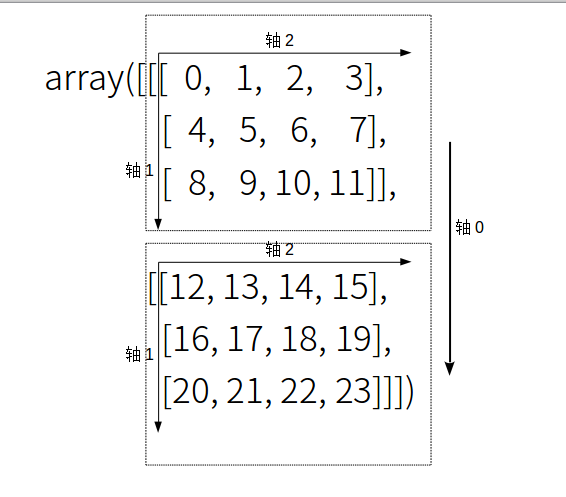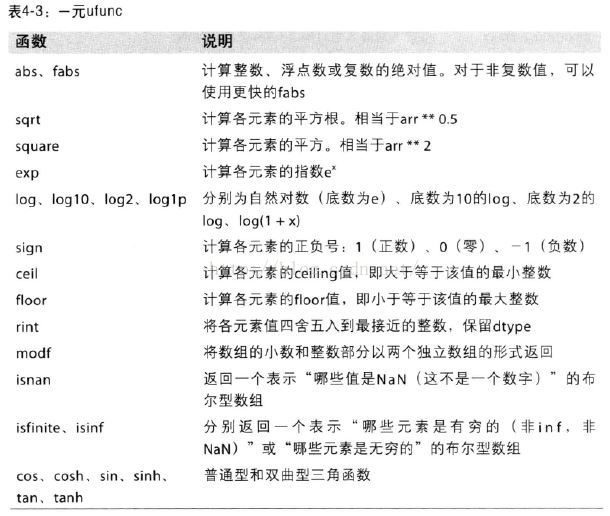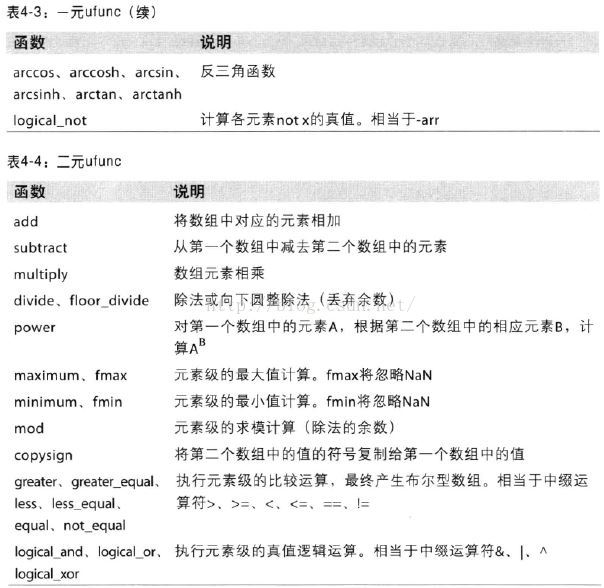• transpose用法：tensor.transpose(a,b) 可以理解为矩阵转置，每次输入两个index，实现转置，是2D的操作 permute用法：tensor.permute(a,b,c) transpose仅可以进行二维转置，而permute则不仅限于二维，可以进行多维度...
transpose用法：tensor.transpose(a,b) 可以理解为矩阵转置，每次输入两个index，实现转置，是2D的操作 permute用法：tensor.permute(a,b,c) transpose仅可以进行二维转置，而permute则不仅限于二维，可以进行多维度转置   举例：
import torch
y = torch.randn(5, 10, 15)
print(y.size())
print(y.view(-1, 15).size())  # Same as doing y.view(50, 15)  view(size) change the size if tensor. if parms is -1 ,then change outomatically
print(y.view(-1, 15).unsqueeze(1).size()) # unsqueeze(index) Adds a dimension at given index.
print(y.view(-1, 15).unsqueeze(1).squeeze().size()) # squeeze() will delete all empty dimension
print('y-size is :', y.size())
print(y.transpose(0, 1).size())#tensor转置,仅限2D，（理解为一次仅能改变两个顺序）
print(y.transpose(1, 2).size())
print(y.transpose(0, 1).transpose(1, 2).size())
print(y.permute(1, 2, 0).size())# better than transpose(), can change more

输出：
torch.Size([5, 10, 15])
torch.Size([50, 15])
torch.Size([50, 1, 15])
torch.Size([50, 15])
y-size is : torch.Size([5, 10, 15])
torch.Size([10, 5, 15])
torch.Size([5, 15, 10])
torch.Size([10, 15, 5])
torch.Size([10, 15, 5])

展开全文permute pytorch
• ## pythontranspose用法

千次阅读 2018-12-22 20:08:26
众所周知，python的numpy模块在数据分析中占有重要的地位，因其所定义的 ndarray （n-dimensional array，多维数组）对象比之python基本类库所定义的 list 对象而言，具有更高的灵活性和更广的适用范围。更重要的...


一、前言
众所周知，python的numpy模块在数据分析中占有重要的地位，因其所定义的 ndarray（n-dimensional array，多维数组）对象比之python基本类库所定义的 list 对象而言，具有更高的灵活性和更广的适用范围。更重要的是，由于numpy模块是用C语言编写的，因此计算机在处理 ndarray 对象时的速度要远快于 list 对象。看一个例子：

>>
import numpy
as np

my_arr = np.arrange(
1000000)

my_list = list(range(
1000000))

>>  %time
for _
in range(
10): my_arr2 = my_arr *
2

CPU times: user
17.7 ms, sys:
12.5 ms, total:
30.1 ms Wall time:
29.9 ms

>>  %time
for _
in range(
10): my_list2 = [x *
2
for x
in my_list]

CPU times: user
525 ms, sys:
105 ms, total:
630 ms Wall time:
629 ms

可以明显地看出，当数字大小达到百万数量级时，使用
ndarray 对象的运算时间比使用
list 对象运算时间有明显的减少。而实际的数据分析中用到的数据只会更大，这时使用
ndarray 显然是一个更为明智的选择。
二、numpy中transpose函数及swapaxes函数的用法

首先从二维数组开始，构造一个数组如下：

>> arr = np.arange(
15).reshape((
3,
5))

arr

array([[
0,
1,
2,
3,
4],

[
5,
6,
7,
8,
9],

[
10,
11,
12,
13,
14]])

reshape的作用是生成一个3X5的二维数组arr。我们可以把此处的arr看作一个3X5阶的矩阵，学过线性代数的朋友应该知道，此时矩阵元素0可表示为a11，7可表示为a23，13可表示为a34。而对应的数组中，0可表示为arr，7可表示为arr，13可表示为arr。
我们可以用arr[m][n]（m=0,1,2;n=0,1,2,3）来表示此数组中的元素。此时我们称此二位数组arr有两条轴，分别表示为0和1，其中m对应的轴为0，n对应的轴为1。对以上数组对应的矩阵进行转置：

>> arr.T

array([[
0,
5,
10]，

[
1,
6,
11],

[
2,
7,
12],

[
3,
8,
13],

[
4,
9,
14]])

相信学过线性代数的朋友很好理解，此矩阵转置操作等价于将轴0和1交换，对应于numpy模块中定义的的transpose函数以及swapaxes函数可表示为：

>> arr.swapaxes(
1,
0)

array([[
0,
5,
10],

[
1,
6,
11],

[
2,
7,
12],

[
3,
8,
13],

[
4,
9,
14]])

>> arr.transpose(
1,
0)

array([[
0,
5,
10],

[
1,
6,
11],

[
2,
7,
12],

[
3,
8,
13],

[
4,
9,
14]])

可以看出，以上变换是等价的，均是一种“轴变换”。
二维数组相对容易理解，三维数组相对复杂一点，但基本原理不变，不过是在二位数组的基础上增加了一条轴。构造以下三维数组：

>> arr = np.arange(
24).reshape((
2,
3,
4))

arr

array([[[
0,
1,
2,
3],

[
4,
5,
6,
7],

[
8,
9,
10,
11]],

[[
12,
13,
14,
15],

[
16,
17,
18,
19],

[
20,
21,
22,
23]]])

此时可用
arr[x][y][z](x=0,1; y=0,1,2; z=0,1,2,3)来表示，此时x对应轴0，y对应轴1，z对应轴2。看下图会更直观一点：由上图可以看出，元素0可表示为arr，元素6可表示为arr，元素12可表示为arr，元素18可表示为arr，元素22可表示为arr。那么此时若使用函数transpose(1,0,2)，即代表将轴0和1对换，轴2不变，亦即将arr[x][y][z]中x和y位置互换，即元素12变为arr，元素22变为arr，以此类推，整个数组将变为：

>> arr.transpose((
1,
0,
2)

array([[[
0,
1,
2,
3],

[
12,
13,
14,
15]],

[[
4,
5,
6,
7],

[
16,
17,
18,
19]],

[[
8,
9,
10,
11],

[
20,
21,
22,
23]]])

同理，swapaxes(1,2)即表示将轴1和2位置互换，轴0不变：

>> arr.swapaxes(
1,
2)

array([[[
0,
4,
8],

[
1,
5,
9],

[
2,
6,
10],

[
3,
7,
11]],

[[
12,
16,
20],

[
13,
17,
21],

[
14,
18,
22],

[
15,
19,
23]]])

相信看到这儿，大家应该对这两个函数的用法更加清楚了吧。
此问题的关键在于搞清楚轴与元素位置的对应关系，以及在进行轴变换时，对应的元素位置会发生怎么样的改变，进而会导致整个数组的形状发生怎样的改变。

tensorflow中： 整个模型是为了算 y=w*X+b中的w，对X进行转置才能让w和X这两个矩阵进行相乘。矩阵w(m,n)和矩阵X(m,n)原本不能相乘，对X进行转置后，矩阵w(m,n)与矩阵X(n,m)就能相乘
转载https://blog.csdn.net/lothakim/article/details/79494782
展开全文• 1、不加参数的情况下，transpose()的作用就是整个矩阵完全置换 import numpy as np A = np.arange(24).reshape(2,3,4) T = A.transpose() print(T.shape) print('______________') print(A) print('_____________...
1、不加参数的情况下，transpose()的作用就是整个矩阵完全置换
import numpy as np

A = np.arange(24).reshape(2,3,4)
T = A.transpose()
print(T.shape)
print('______________')
print(A)
print('______________')
print(T)
print('______________')
print(A[1,2,3])
print(T[3,2,1])
output：
(4, 3, 2)
______________
[[[ 0  1  2  3]
[ 4  5  6  7]
[ 8  9 10 11]]

[[12 13 14 15]
[16 17 18 19]
[20 21 22 23]]]
______________
[[[ 0 12]
[ 4 16]
[ 8 20]]

[[ 1 13]
[ 5 17]
[ 9 21]]

[[ 2 14]
[ 6 18]
[10 22]]

[[ 3 15]
[ 7 19]
[11 23]]]
______________
23
23
2、加参数时，必须根据矩阵的维度来设置参数
import numpy as np

#对于该矩阵可以认为有三个维度，即0,1,2
A = np.arange(24).reshape((2,3,4))
print(A)
#如果不改变原矩阵，那么正常的参数顺序是：(0,1,2)
T = A.transpose(0,1,2)
print(T)
print('_____________________')
#如果想要置换第1和第2个维度，则参数顺序为：(1, 0 ,2)
T = A.transpose(1,0,2)
print(A[0,1,2])
print(T[1,0,2])
print('_____________________')
#如果要置换第1和第3个维度，则参数的顺序为：(2,1,0)
T = A.transpose(2,1,0)
print(A[0,1,2])
print(T[2,1,0])
output：
[[[ 0  1  2  3]
[ 4  5  6  7]
[ 8  9 10 11]]

[[12 13 14 15]
[16 17 18 19]
[20 21 22 23]]]
[[[ 0  1  2  3]
[ 4  5  6  7]
[ 8  9 10 11]]

[[12 13 14 15]
[16 17 18 19]
[20 21 22 23]]]
_____________________
6
6
_____________________
6
6


展开全文• ## pythontranspose

千次阅读 2017-03-02 18:43:23
在读图片时，会用到这么的一段代码： image_vector_len = np.prod(image_size)#总元素大小，3*55*47 img = Image.open(path)  arr_img = np.asarray(img, dtype='float... arr_img = arr_img.transpose(2,0,1).re
1.数组转置和轴对换：数组不仅有transpose方法，还有一个特殊的T属性：
arr = np.arange(15).reshape(3,5)
arr输出：
array([[ 0,  1,  2,  3,  4],
[ 5,  6,  7,  8,  9],
[10, 11, 12, 13, 14]])
arr.T  输出：
array([[ 0,  5, 10],
[ 1,  6, 11],
[ 2,  7, 12],
[ 3,  8, 13],
[ 4,  9, 14]]) 2.进行矩阵计算时，经常需要用到该操作，比如利用
np.dot计算矩阵内积XTX：

    arr = np.random.randn(6,3)
arr  
输出：
array([[-0.83790345, -1.13304154, -0.42567014],
[ 0.75742538,  1.24634357, -1.00116761],
[ 0.54168995, -0.83717253, -1.11580943],
[-0.13315165,  0.0331654 ,  0.70605975],
[-2.57536154, -0.68951735,  1.16959181],
[-1.26193272, -1.24703158,  0.3183666 ]])
np.dot(arr.T,arr) 输出：
array([[ 9.81189403,  4.78491411, -4.51395404],
[ 4.78491411,  5.56963513, -1.01142215],
[-4.51395404, -1.01142215,  4.39638499]]) 3.对于高维数组，
transpose需要得到一个由轴编号组成的元组才能对这些轴进行转至（比较难理解）：
arr = np.arange(16).reshape((2,2,4))
arr 
输出：
array([[[ 0,  1,  2,  3],
[ 4,  5,  6,  7]],

[[ 8,  9, 10, 11],
[12, 13, 14, 15]]])
输出：array([[[ 0,  1,  2,  3],
[ 8,  9, 10, 11]],

[[ 4,  5,  6,  7],
[12, 13, 14, 15]]])提示：transpose(1,0,2)把原来的shape由(2,2,4)变成了(2,2,4)，就是第一个轴和第二个轴上面的元素互换。比如原来位置（0,1,0）上的元素为4，现在把它放到了（1,0,0）这个位置，就是下面那个位置由8变成了4，标出了红色。arr.transpose((1,0,2))  4.ndarray还有一个swapaxes方法，它接受一对轴变换：arr 输出：array([[[ 0,  1,  2,  3],
[ 4,  5,  6,  7]],

[[ 8,  9, 10, 11],
[12, 13, 14, 15]]])arr.swapaxes(1,2) 输出：array([[[ 0,  4],
[ 1,  5],
[ 2,  6],
[ 3,  7]],

[[ 8, 12],
[ 9, 13],
[10, 14],
[11, 15]]])5.通用函数sqrt、exp、maximum：arr = np.arange(10)
arr 输出：array([0, 1, 2, 3, 4, 5, 6, 7, 8, 9])np.sqrt(arr) 输出：array([ 0.        ,  1.        ,  1.41421356,  1.73205081,  2.        ,
2.23606798,  2.44948974,  2.64575131,  2.82842712,  3.        ])np.exp(arr)  输出：array([  1.00000000e+00,   2.71828183e+00,   7.38905610e+00,
2.00855369e+01,   5.45981500e+01,   1.48413159e+02,
4.03428793e+02,   1.09663316e+03,   2.98095799e+03,
8.10308393e+03])x = np.random.randn(8)
x  输出：array([-0.24726724,  0.69709717,  0.9658356 ,  1.89019088, -0.28912795,
-0.09235779,  0.37690775,  0.9102138 ])y = np.random.randn(8)
y  输出：array([-0.05048326, -0.02207697, -0.59940773, -1.32029941,  0.30894105,
-0.05807405, -1.5019804 ,  0.12918562])np.maximum(x,y) #元素级最大值  输出：array([-0.05048326,  0.69709717,  0.9658356 ,  1.89019088,  0.30894105,
-0.05807405,  0.37690775,  0.9102138 ])6.modf函数可以把数组分别提取出整数部分和小数部分arr = np.random.randn(7)*5
arr输出：array([ -1.53462646,   6.15168006,   4.32588912,  -0.05408803,
-2.98953481, -10.83013834,   1.13673478])np.modf(arr) 输出：(array([-0.53462646,  0.15168006,  0.32588912, -0.05408803, -0.98953481,
-0.83013834,  0.13673478]),
array([ -1.,   6.,   4.,  -0.,  -2., -10.,   1.]))部分一元、二元函数总结如下：展开全文• ## pythontranspose的理解

千次阅读 2017-04-03 22:26:30
网上看到这样一个问题，研究了一下， import numpy as np x = np.arange(24).reshape((2,3,4)) ...print(x.transpose((1,0,2))) # shape(3,2,4) [[[ 0 1 2 3] [ 4 5 6 7] [ 8 9 10 11]] [[12 13 14 15] [16 17
• ## Python numpy.transpose 详解

万次阅读 多人点赞 2017-12-27 15:20:44
前言看Python代码时，碰见 numpy.transpose 用于高维数组时挺让人费解，通过一番画图分析和代码验证，发现 transpose 用法还是很简单的。 正文Numpy 文档 numpy.transpose 中做了些解释，transpose 作用是改变序列，...numpy
• transpose 的原理其实是根据维度（shape）索引决定的，举个栗子： x = np.arange(4).reshape((2,2)) //生成一个2x2的数组 print(x) [[0 1] [2 3]] 我们生成了一个维度为二维的数组，其中有两个索引值（矩阵的行与...
• Numpy numpy.transpose(),可以实现矩阵的转置。 也可以输入参数，实现行与列的转置 # making a 3x3 array a = np.array([[1, 2], [4, 5], [7, 8]]) # before transpose print(a, end ='\n\n') # after ...
• >>> a = array([[[ 0, 1, 2, 3], [ 4, 5, 6, 7]], [[ 8, 9, 10, 11], [12, 13, 14, 15]]]) >>> b = a.transpose(１，０，２) array([[[ 0, 1, 2, 3],
• transpose是什么意识呢？ 看如下例子：arr1 = np.arange(16).reshape((2,2,4)) arr1array([[[ 0, 1, 2, 3], [ 4, 5, 6, 7]], [[ 8, 9, 10, 11], [12, 13, 14, 15]]])这是原来的矩阵。如果对其进行转置，...
• Python的Numpy库的时候，被 numpy.transpose 函数用于高维数组搞的一头雾水，不明白原理，通过手动分析和代码验证，发现 transpose 的具体用法。 transpose 作用是改变序列，下面是一些小例子： 在不添加参数的...
• python transpose() 转载于:https://www.cnblogs.com/bafenqingnian/p/8706754.html
• 首先看一个transpose多维的转置例子In :arr = np.arange(16).reshape(2,2,4) In :arr.transpose((1,0,2)) Out: array([[[ 0, 1, 2, 3], [ 4, 5, 6, 7]], [[ 8, 9, 10, 11], [12, 13, 1Numpy
• 初学python,在看别人写的代码的时候遇到复数表示的维度序号，就让我感到有点疑惑，用代码尝试了几次，还是没有发现其中的规则。例如有四维的tenson,形状是[3, 4,2, 1], transpose(-1, 1)表示哪两个维度进行转换有问必答
• * for in 嵌套列表 ...def transpose1(matrix): cols = len(matrix) return [[row[i] for row in matrix] for i in range(0,cols)] def transpose2(matrix): transposed = [] for i in range(len(ma...
• 转置有三种方式，transpose方法、T属性以及swapaxes方法。 1 . T 适用于一、二维数组 In : import numpy as np In : arr = np.arange(20).reshape(4,5)#生成一个4行5列的数组 In : arr Out: ...
• Python代码时，碰见 numpy.transpose 函数用于高维数组时不明白原理，通过一番画图分析和代码验证，发现 transpose 用法可以清晰地介绍给大家。 transpose 作用是改变序列，下面是一些小例子： 代码1： x = np...
• 利用C++实现矩阵转置.其中矩阵的维数可以按照实际需要进行增加，代码只是简单的进行了初始化。
• 因本人刚开始写博客，学识经验有限，如有不正之处望读者指正，不胜...这是关于Python3中numpy.transpose()用法的详解。 关于对numpy.transpose()函数的清晰理解： 转载链接：详解numpy中transpose()函数 transpos...
• 文章“Python numpy.transpose 详解”（https://blog.csdn.net/u012762410/article/details/78912667 ）中，对transpose的原理进行了解释，但在阅读过程中，发现该文的图片有误，故现保留其原文文字部分不变，仅对其...axis 变换
• 这问题让我想到了48题，应该非常简单，或者说都没必要在这里写，我主要的目的是总结一下Python中的 zip 的用法哈。 Python3实现： # @Time :2018/7/9 # @Author :LiuYinxing class Solution: def ...zip 算法 LeetCode
• 一、前言众所周知，python的numpy模块在数据分析中占有重要的地位，因其所定义的 ndarray（n-dimensional array，多维数组）对象比之python基本类库所定义的 list 对象而言，具有更高的灵活性和更广的适用范围。...numpy array
• 然后我们在看一下transpose（a,b,c）是怎么运行的，又得到什么结果。 首先是transpose（0，1, 2） 完全没有变化 然后是tranpose(0,2,1) 变化有点大啊，条换了b和c的位置就有这样 的结果，这是为啥呢？ ...
• transpose函数 numpy.transpose (arr, axes) transpose： 反转或排列数组的轴； 返回修改后的数组；对于具有两个轴的数组a，transpose(a)给出矩阵转置. 参数 arr：要操作的数组 axes(可选)：整数或列表（如果指定...numpy
• np.transpose(a,axis=None) 输入：1.阵列array a 2.转置的轴 输出：返回 转置后的 阵列array 把矩阵的第一个方括号“[]”为 0轴 ，第二个方括号为 1轴 ，第三个方括号为 2轴 这种思维方式有利于本函数分析，参见 ......

# pythontransposepython 订阅